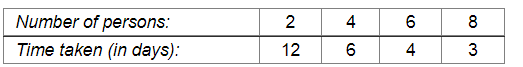# The following table gives the information regarding the number of

Question:

The following table gives the information regarding the number of persons employed to a piece of work and time taken to complete the work:Plot a graph of this information.

Solution:

Here, number of persons is an independent variable and time taken is a dependent variable. So, we take the number of persons on the x-axis and time taken on the y-axis.

Let us choose the following scale:

On x-axis: 2 cm = 2 persons

On y-axis: 2 cm = 2 days

Now, let us plot (2, 12), (4, 6), (6, 4), (8, 3). These points are joined to get the graph representing the given information as shown in the figure below.​